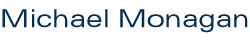My research area is known as computer algebra. I have worked on the Maple project since 1983. Maple is one of several computer algebra systems. It was developed at the University of Waterloo in the 1980s. I can supervise graduate students at Simon Fraser University who are interested in pursuing a graduate degree in computer algebra at the Ph.D. and Masters' level. Students need to have an interest in both mathematics and computer science. I am currently looking for a PhD student to start Fall 2019. I am looking for a student who loves mathematics and loves programming and has programming experience in C or C++. I would like the student to help me develop a library of algebraic algorithms for operations with polynomials in n variables. The focus of the library will be on algorithm for sparse polynomial interpolation and algorithms that use sparse polynomial interpolation. I also want to develop parallel algorithms. No parallel programming experience is necessary. Before you send me an application, please find out what computer algebra is, and in your letter, say why you are interested in doing computer algebra, and what programming experience you have.

For details of my current research projects, please see my recent publications.

Isaac Jinadu, Ph.D. student in pure mathematics, in progress.
Kim Connolley, MSc. student in pure mathematics, in progress.
Tian Chen, Ph.D. student in applied mathematics, in progress.
Garrett Paluck, MSc. in pure mathematics, in progress.
Mahdi Javadi. M.Sc. in computer science, November 2006.
• Thesis: A Sparse Modular GCD Algorithm for Polynomials over Algebraic Function Fields.
Aaron Bradford. M.Sc. in pure mathematics, April 2006.
• Project: Computing Discrete Logarithms in GF(p).
Allan Wittkopf. Ph.D. in applied and computational mathematics, October 2004.
• Thesis: Algorithms and Implementations for Differential Elimination.
Stephen Tse. M.Sc. in computing science, July 2002.
• Thesis: Algorithms and Bounds for Resultants.
Jennifer de Kleine. M.Sc. in computing science, 2001.
• Project: A Modular Design and Implementation of Buchberger's Algorithm.
Laurent Bernardin. Ph.D. in computer science, ETH Zentrum, September 1999.
• Thesis: Factorization of Multivariate Polynomials over Finite Fields.

Robyn Hearn, Big Data, 2018.
• Roots of Polynomials.
Ryan Mehregan, NSERC 2018.
• Hensel Lifting.
Gabriel Henderson, NSERC 2017.
• Sparse Interpolation.
Jesse Elliott, NSERC 2016.
• Sparse Interpolation.
Alex Fan, RA 2016.
• Sparse Interpolation.
• Discrete Logarithms.
Hao Zhuang, USRA 2015.
• FFT over Finite Fields.
Marshall Law, NSERC 2014.
• FFT multiplication mod p in parallel.
Casie Bao, VPR 2014.
• Measuring river flow (discharge).
Mathew Gibson, NSERC 2013.
• Algorithms for GCDs over finite fields.
Alan Wong, NSERC 2013.
• Computing Tutte polynomials.
• Algorithms for finite groups.
• Fast polynomial arithmetic.
Stephen Melczer, NSERC 2010.
• Algebraic geometry.
Julian Sahrasbuhde, NSERC 2009.
• Graph theory.
Bill Bao, NSERC 2009.
• Scientific computing.
Asif Zaman, NSERC 2008.
• Computational group theory.
Andrew Arnold, NSERC 2007.
• Graph theory and number theory.
Simon Lo, NSERC, 2006.
• Graph theory.
Al Erickson, NSERC, 2006.
• Graph theory and polynomial factorization.
Simon Lo, NSERC, 2005.
• Computer algebra.
Howard Liu, NSERC, 2005.
• Numerical integration and differentiation.
Al Erickson, NSERC, 2005.
• Computer algebra.
Simon Lo, NSERC, 2004.
• Computational linear algebra.
Mohammed Ebrahimi, NSERC, 2004.
• Visualizations for differential equations in Maple.
Scott Cowan, NSERC, 2003.
• Computational problems in cryptography and algebra.
• Evaluating definite integrals.
Roman Pearce, NSERC, 2001.
• Groebner bases and ideal theoretic operations.
George Zhang, NSERC, 2001.
• Algorithms for testing ideals for primality and maximality.
Jamie Mulholland, NSERC, 2001
• Algorithms for trigonometric polynomials.
Craig Pastro, NSERC, 2000.
• The Modular GCD algorithm over algebraic number fields.
Michael Ludkovski, Directed Studies, 1999.
• Brown's and Zippel's modular GCD algorithms.
Mark Siggers, Directed Studies, 1999
• Univariate Hensel lifting over Euclidean domains.
Colin Percival, Career Prep, 1998.
• Polynomial GCDs over algebraic number fields.
Rene Rodoni, Diplomarbeit, ETH Zurich, 1995.
• An Implementation of the Forward adn Reverse Mode in Maple.
Roger Margot, Diplomarbeit, ETH Zurich, 1995.
• Univariate polynomial GCD's over Q(alpha).
Igor Berchtold, Diplomarbeit, ETH Zurich, 1993.
• Sparse Matrix Determinants over Integral Domains.
Laurent Bernardin, Diplomarbeit, ETH Zurich, 1993.
• Factorization of multivariate polynomials over a finite field.
Walter Neuenschwander, Diplomarbeit, ETH Zurich, 1992.
• Algorithmische Differentiation.
Stefan Schwendimann, Diplomarbeit, ETH Zurich, 1992.
• Ein Softwarepaket fuer die algebraische, projektive Geometrie.

p. (778) 782-4279 · Shrum Science K 10501 · Department of Mathematics · Simon Fraser University · 8888 University Drive · Burnaby · BC · V5A 1S6 · Canada